Next: Superposition Up: State Vectors and Dirac Previous: State Vectors:   Contents

#### Dirac Notation:

The standard notation for a state vector is a ket vector |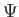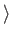. For example, a vector in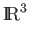with basis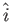,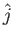,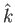is typically written as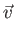= a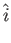+ b+ c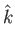= (a, b, c)T.

In Dirac notation, it would be written as

| v= a| i+ b| j+ c| k= (a, b, c)T.

The term ket and this notation come from the physicist Paul Dirac who wanted a concise way of writing formulas involving row and column vectors. He referred to row vectors as bra vectors represented as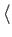y|. The inner product of a bra and a ket vector is writteny| x, and is called a bracket .Next: Superposition Up: State Vectors and Dirac Previous: State Vectors:   Contents
Matthew Hayward Lower Query Bounds in the Quantum Oracle Model GitHub Repository